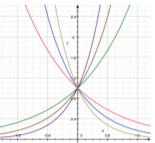Exponential FunctionsHenri Picciotto

The first two activities are intended for grades 10-11 (Algebra 2, or perhaps Precalculus.)

Rolling Dice

You may print and duplicate the worksheet (TI-83, Fathom, Geogebra).
If you create a Desmos version of the worksheet, send it to me. I'll post it and acknowledge you.

This is an experimental model of exponential decay. It requires the use of large numbers of ten-sided dice, which can be purchased at games stores. This is a bit expensive, but worth it, given the use in this lab and in many labs about probability.

This lab is a wonderful illustration of a situation where the rate of change of a quantity is proportional to that quantity, though I don't usually discuss this with my Algebra 2 students. What we do discuss is the relationship between the theoretically expected points, and the ones obtained experimentally. When they see the theoretical curve passing so close to the experimental points on the screen, students are invariably amazed at how well the model fits the reality.

These Fathom files help calculate the class averages: four groups, seven groups.

Exponential Graphs

Graphing a given function by hand by plotting points and connecting them is tedious, and for most students does not entail a lot of learning. Electronic graphing has a place, of course, but again, for most students it does not entail a lot of learning, unless an activity is carefully designed to yield some understanding.

These worksheets, in contrast, force thinking and discussion about the graphs of exponential functions, and (perhaps with some guidance from the teacher) they should lead to some awareness and understanding of key features of the functions and their graphs: whether they are increasing or decreasing, the y-intercept, the image of 1, and the relationship between the equations of graphs that are reflections of each other. The worksheets also present an opportunity to discuss negative exponents.

Exponential Graph Similarity

This activity is intended for teachers, but may work well in a post-Algebra 2 elective course. Prerequisites: some understanding of geometric transformations, some facility with GeoGebra or other interactive geometry software.

Exponential graphs are not usually considered to be geometric objects. However, you might find it interesting that all exponential graphs are similar. Intrigued? Download the worksheet.